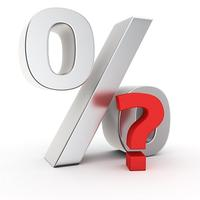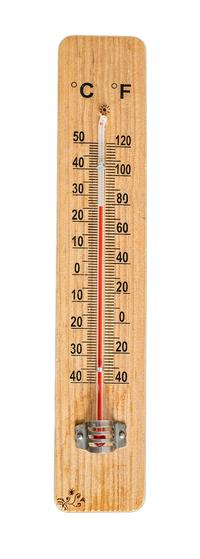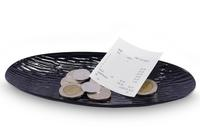# Maths: life hacks## Percentages

x% of y = y% of xThis might look a little complicated with all the symbols, but what it really says is that you can work out a percentage in whatever order is easiest. For example, 4% of 75 is the same as 75% of 4, which we know to be 3.

The reason it works is due to the multiplications involved when doing a percentage calculation. Taking the example above: for 4% of 75 we are really working out 4/100 * 75. Because the order in which we multiply or divide numbers doesn’t matter (commutativity is the technical term for this), we can instead rearrange this as 75/100 * 4, which is exactly how we would calculate 75% of 4. Therefore, we get the same answer!

My short social media video helps explain this trick.

## Temperature conversions: changing from Fahrenheit to CelsiusFortunately, there aren’t many countries around the world still using the Fahrenheit system, but for the few that still do (here’s looking at you America), it can be a real pain trying to work out whether or not you need your coat when heading out for the day.

But not to worry – there’s a simple trick you can use to get a pretty accurate estimate when converting from Fahrenheit to Celsius: subtract 30 and divide by two. Here are a few examples:

100o F = (100 – 30)/2 = 70/2 = 35o C    [actual value 38o C]

60o F = (60 – 30)/2 = 30/2 = 15o C        [actual value 16o C]

30o F = (30 – 30)/2 = 0/2 = 0o C           [actual value -1o C]

So, whilst not perfect, it certainly gives you a very good idea of whether or not to bring your coat… (For anyone looking for the exact formula, subtract 32 and multiply by 5/9).

My short social media video explains how to convert the other way around – from Celsius to Fahrenheit.

## TippingWhen eating out in the UK, chances are you’re either not expected to tip, or if you do, it’s a nice and simple 10%. To calculate a 10% tip just take the total and move the decimal point one place to the left. For example, on a bill of £65.10, moving the decimal point one place to the left gives a 10% tip of £6.51.

Now in the US, things aren’t quite so simple – I’ve seen everything from 12.5% to 15%, 17%, 17.5%, 18%, 20%... But, there are a few tricks you can use to help make the calculations easier.

A good starting point is often to use the same 10% trick as described above, ie move the decimal point one place to the left. The reason it’s helpful to start out knowing 10% is that this can then be halved (and if needed halved again) to give you 5% (and 2.5% respectively). Let’s take a \$88.40 bill as an example:

10% = \$8.84   by moving the decimal point one place to the left

5% = \$4.42     by halving the 10% value

2.5% = \$2.21  by halving the 5% value

Once we have these key values, we can now calculate a 12.5%, 15% or 17.5% tip by adding together the required amounts. You still need to do some addition of course, but it’s certainly a lot easier than trying to do 17.5% of \$88.40 right from the off!

A 20% tip works very similarly, with the 10% value now just needing to be doubled. So here, that will give a 20% tip of two times \$8.84, which gives \$17.68.

Finally, for the trickier 17% or 18% tips we go back to the 10% value and use the same method of moving the decimal point to the left to calculate 1% of the total. Doing this twice from our original value of \$88.40 gives \$0.88, which is equal to 1%. Now, just as before, we can add the required number of 1% values to the 15% tip until we get to the desired 17% or 18% tip.

You could of course do all of this on your phone calculator, but what better way to practise your mental arithmetic and also impress your friends at the same time!

Dr Tom Crawford | St Edmund Hall | @tomrocksmaths